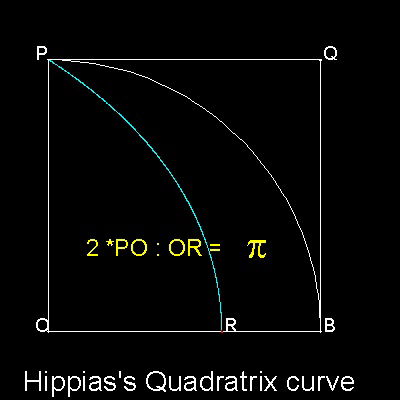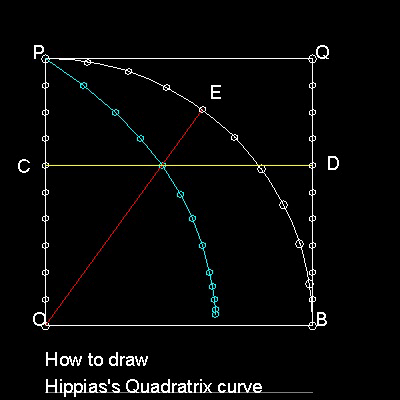Squaring the Circle
Go to   Fun_Math Content Table   Three Famous Problems   Greek Circle Squarer

### Greek Circle SquarersDinostratus (about 390 BC- about 320 BC), a brother of Menaechmus (about 380 BC - about 320 BC), gave a proof for the following proposition using "reductio ad absurdum".

(arc PB) : PO = PO : OR

Or OR = (2/p) PO

For detail of the proof, see ref.1.

The modern derivation of this relation is given in the next section .
******* Hippias_circle_squarer_desc.dwg *******

#### How to Draw a QuadratrixOBQP is a square.

Divide both OP and BQ in equal parts N.

Divide a quarter circle BP into the same number N. Point C,D & E arer such points.

Horizontal line CD(yellow) and polar line OE(red) intersect .

The locus of such points(cyan color) is the "Quadratrix".

You can see the process in animation.

To create this drawing and animation:
Then from command line, type quadratrix_2 for drawing quadratrix for 1000 division.
test_1  & test_2   for drawing manually.

If OP & OB are x & y axis respectively, the curve is expressed as y = x tan(p*y/2)

Using identity tan(a) = sin(a)/cos(a), and replacing (p/2)y = h

x = (2/p)*cos(h)*(h/sin(h))

As h approaches zero, both cos(h) and (h/sin(h)) approach 1.

So the x-coordinate of the point R,where the Quadratrix intersect X-axis is (2/p).

This means that length OR is used to get p value, and we now have succeeded in "Squaring the Circle".
This is the reason why this curve is named "Quadratrix", i.e. curve for circle quadrature.

#### References

Go to   Fun_Math Content Table   Three Famous Problems   Greek Circle Squarer

All questions/suggestions should be sent to Takaya Iwamoto

Last Updated Jan 22, 2007